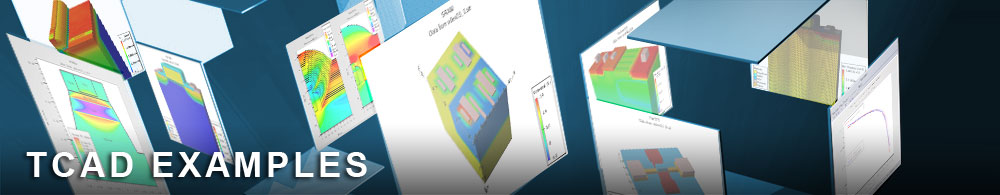Breakdown Simulation with EB and NEB Models

## diodeex02.in : Breakdown Simulation with EB and NEB Models

Requires: S-Pisces/Giga
Minimum Versions: Atlas 5.28.1.R

This example demonstrates breakdown simulation of a 1D diode, including second breakdown, with Energy Balance (EB) and Nonisothermal Energy Balance (NEB) Models.

Breakdown simulation for submicron devices should be simulated using the Energy Balance Model due to nonlocal impact ionization effects, which can substantially influence device characteristics. For high current levels the thermal self-heating effects can also play an important role by decreasing the mobility and impact ionization rate. This example demonstrates a comparison of breakdown calculations obtained with Energy Balance and Nonisothermal Energy Balance Models.

The first Atlas run uses the Energy Balance Model: Poisson's equation, carrier continuity equations, and energy balance equation for electrons and holes are solved self-consistently.

In the first part of the input file the device is described, including mesh, electrodes locations, and doping distribution. The material statement is then used to assign energy relaxation times for electrons and holes. The models statement is used to select a set of physical models for this simulation. In this case, these models are SRH and AUGER recombination, the concentration and field dependent mobility model, band gap narrowing, and energy balance equations for electrons and holes (hcte). The impact statement is used to assign the energy relaxation lengths for the Selberherr model. The contact statement is used to specify a large resistor at the emitter electrode, providing a smooth transition from voltage boundary conditions to current boundary conditions. It is not needed if the curve tracing algorithm is used (see the following example).

The numerical methods used in EB simulation can strongly affect convergence and CPU time. Here method block newton is used. This decouples the carrier temperature calculation from the potential and continuity equations at lower biases. This allows for larger bias steps at low bias.

The initial biasing is done by ramping the emitter contact towards 25V. A compliance limit is set on the emitter current as defined by: cname=emitter compl=<value> . Once this current is exceeded the voltage ramp stops and Atlas proceeds to the next simulation line. This is to specify current forcing on the emitter contact. The syntax contact name=emitter current does this.

After setting the newton method the biasing proceeds by specifying istep=<value> imult . The parameter imult indicates that the istep is a multiplier to the current as opposed to an additive step. Therefore at each bias step the forced current is multiplied by istep. ifinal indicates the maximum current to be simulated.

Note that due to the use of current forcing, emitter int. bias should be used as the x axis on the IV data plots.

The second Atlas run uses the Nonisothermal Energy Balance Model: Poisson's equation, carrier continuity equations, energy balance equation for electrons and holes, and the lattice heat flow equation are solved self-consistently.

The same set of models is used, except that the solution of the lattice energy balance equation is activated using the models lat.temp statement.

In addition, the thermal boundary conditions should be defined in this case. Thermal boundary conditions are defined in the thermcontact statement. Values of the thermal conductances are specified at the thermal contact located along the emitter and base electrodes.

The results of the two simulation runs are then overlaid using TonyPlot.

To load and run this example, select the Load button in DeckBuild > Examples. This will copy the input file and any support files to your current working directory. Select the Run button in DeckBuild to execute the example.